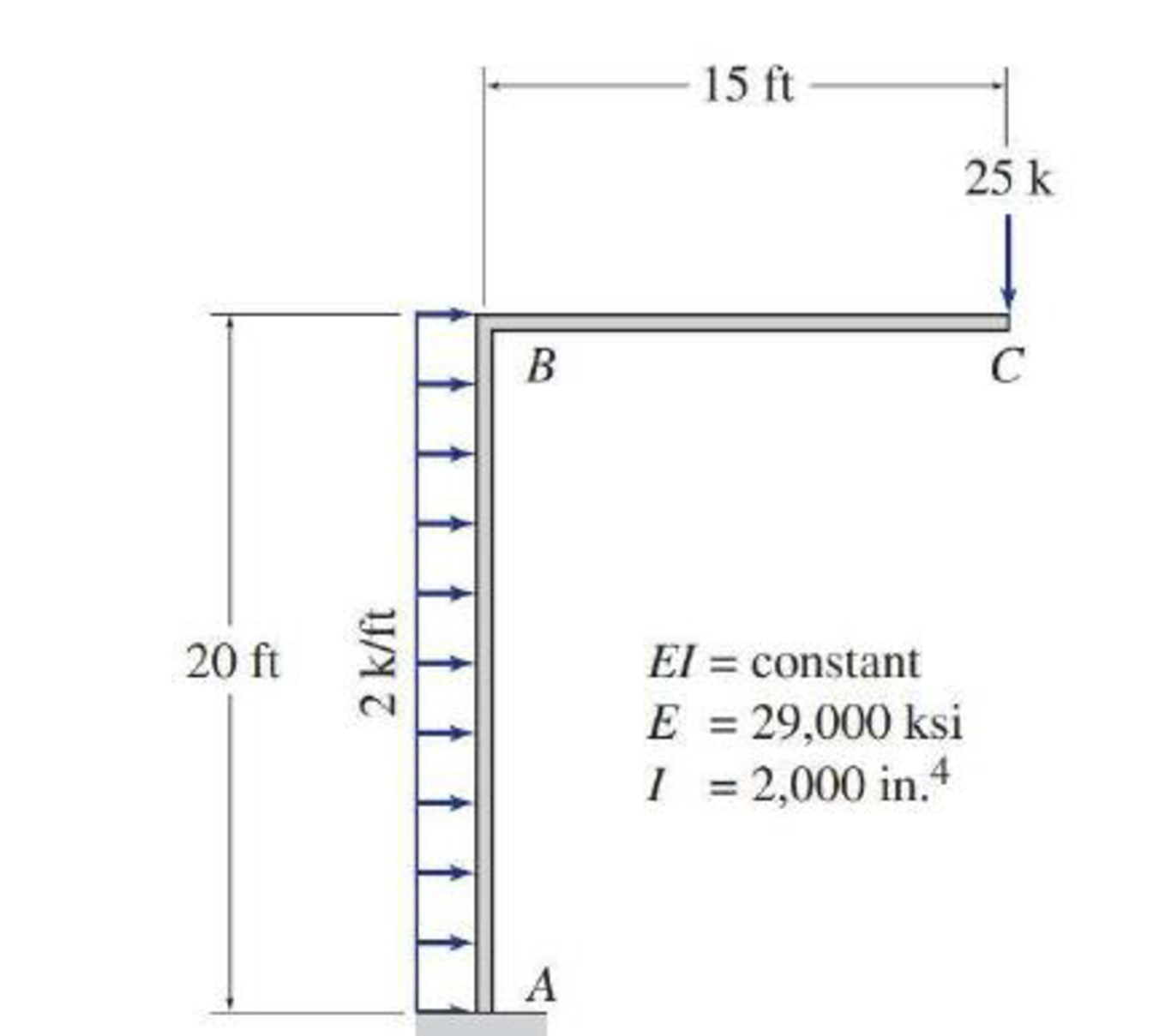# Use Castigliano’s second theorem to determine the vertical deflection at joint C of the frame shown in Fig. P7.36.

#### Solutions

Chapter
Section
Chapter 7, Problem 65P
Textbook Problem
439 views

## Use Castigliano’s second theorem to determine the vertical deflection at joint C of the frame shown in Fig. P7.36.To determine

Find the vertical deflection at joint C of the frame using Castigliano’s second theorem.

### Explanation of Solution

Given information:

The frame is given in the Figure.

The value of E is 29,000 ksi and I is 2,000in.4.

Apply the sign conventions for calculating reactions, forces and moments using the three equations of equilibrium as shown below.

• For summation of forces along x-direction is equal to zero (Fx=0), consider the forces acting towards right side as positive (+) and the forces acting towards left side as negative ().
• For summation of forces along y-direction is equal to zero (Fy=0), consider the upward force as positive (+) and the downward force as negative ().
• For summation of moment about a point is equal to zero (Matapoint=0), consider the clockwise moment as negative and the counter clockwise moment as positive.

Calculation:

Let apply a load P at joint C in the desired direction to find the deflection.

The value of load P is 25 k.

Sketch the frame with load P as shown in Figure 1.

Let the equation for bending moment at distance x in terms of load P be M, the derivative of M with respect to P is MP.

Find the reactions and moment at the supports A:

Summation of moments about A is equal to 0.

MA=0MAP(15)2(20)(202)=0MA15P(15)400=0MA=400+15P

Summation of forces along y-direction is equal to 0.

+Fy=0AyP=0Ay=P

Summation of forces along x-direction is equal to 0.

+Fx=0Ax+2(20)=0Ax=40k

Find the equations for M and MP for the 2 segments of the frame as shown in Table 1.

 Segment x-coordinate M ∂M∂P Origin Limits (ft) BA A 0−20 (400+15P)−40x+2(x)(x2) 15 CB C 0−15 −Px −x

Substitute the value of load P as 25 in the Column 4 of Table 1.

The expression for deflection at C using Castigliano’s second theorem (ΔC) is shown as follows:

ΔC=0L(MP)MEIdx (1)

Here, L is the length of the beam.

Rearrange Equation (1) for the limits 020 and 015 as follows.

ΔC=1EI[020(MP)Mdx+015(MP)Mdx]

Substitute 15 for MP, (400+15(25))40x+x2 for M for the limits 020, x for MP, and 25x for M for the limits 015

### Still sussing out bartleby?

Check out a sample textbook solution.

See a sample solution

#### The Solution to Your Study Problems

Bartleby provides explanations to thousands of textbook problems written by our experts, many with advanced degrees!

Get Started

Find more solutions based on key concepts
Find the equation that best fits the following set of data points. Compare the actual and predicted y values. P...

Engineering Fundamentals: An Introduction to Engineering (MindTap Course List)

What is an entity supertype, and why is it used?

Database Systems: Design, Implementation, & Management

On which networking device do you configure VLANs?

Network+ Guide to Networks (MindTap Course List)

Who is ultimately responsible for the security of information in the organization?

Principles of Information Security (MindTap Course List)

Explain why modern hydraulic braking systems are dual designs, and why this is important.

Automotive Technology: A Systems Approach (MindTap Course List)

What s the Hawthorne Effect? Have you ever experienced it? When and where?

Systems Analysis and Design (Shelly Cashman Series) (MindTap Course List)